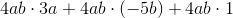## Example Questions

### Example Question #1 : How To Multiply A Monomial By A Polynomial

Multiply: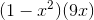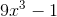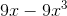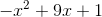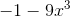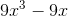Explanation:

Distribute the monomial through the polynomial.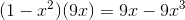The answer is:### Example Question #2 : How To Multiply A Monomial By A Polynomial

Evaluate: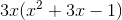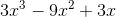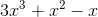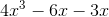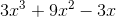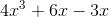Explanation:

Distribute the monomial through each term inside the parentheses.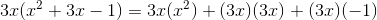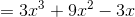### Example Question #3 : How To Multiply A Monomial By A Polynomial

Multiply the monomials: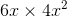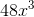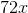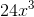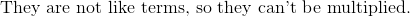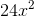Explanation:

In order to multiply this, we can simply multiply the coefficients together and thevariables together.  Anytime we multiply a variable of the same base, we can add the exponents.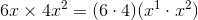Simplify the right side.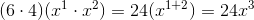The answer is:### Example Question #4 : How To Multiply A Monomial By A Polynomial

Distribute: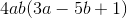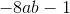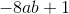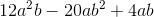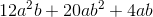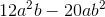Explanation:

Distribute the monomial to each part of the polynomial, paying careful attention to signs: